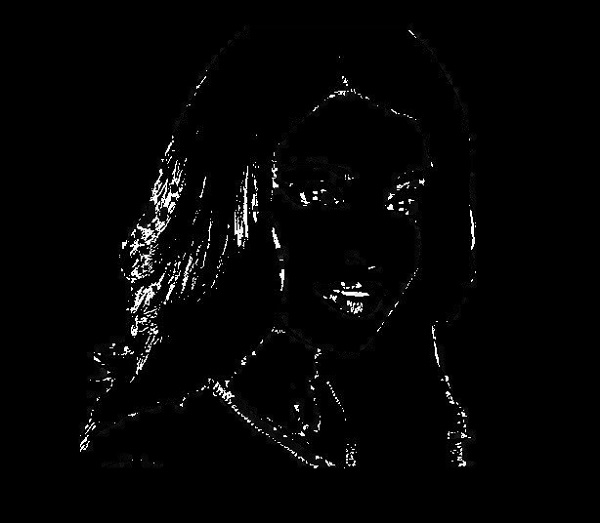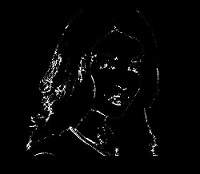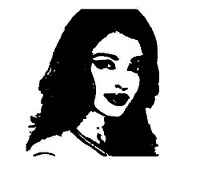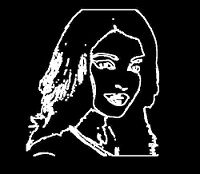# OpenCV - Morphological Operations

In the earlier chapters, we discussed the process of erosion and dilation. In addition to these two, OpenCV has more morphological transformations. The morphologyEx() of the method of the class Imgproc is used to perform these operations on a given image.

Following is the syntax of this method −

```morphologyEx(src, dst, op, kernel)
```

This method accepts the following parameters −

• src − An object of the class Mat representing the source (input) image.

• dst − object of the class Mat representing the destination (output) image.

• op − An integer representing the type of the Morphological operation.

• kernel − A kernel matrix.

### Example

The following program demonstrates how to apply the morphological operation "top-hat" on an image using OpenCV library.

```import org.opencv.core.Core;
import org.opencv.core.CvType;
import org.opencv.core.Mat;
import org.opencv.imgcodecs.Imgcodecs;
import org.opencv.imgproc.Imgproc;

public class MorphologyExTest {
public static void main(String args[]) {

// Reading the Image from the file and storing it in to a Matrix object
String file ="E:/OpenCV/chap12/morph_input.jpg";

// Creating an empty matrix to store the result
Mat dst = new Mat();

// Creating kernel matrix
Mat kernel = Mat.ones(5,5, CvType.CV_32F);

// Applying Blur effect on the Image
Imgproc.morphologyEx(src, dst, Imgproc.MORPH_TOPHAT, kernel);

// Writing the image
Imgcodecs.imwrite("E:/OpenCV/chap12/morph_tophat.jpg", dst);

System.out.println("Image Processed");
}
}
```

Assume that following is the input image morph_input.jpg specified in the above program.### Output

On executing the program, you will get the following output −

```Image Processed
```

If you open the specified path, you can observe the output image as follows −## More Operations

In addition to the morphological operation TOPHAT, demonstrated in the previous example, OpenCV caters various other types of morphologies. All these types are represented by predefined static fields (fixed values) of Imgproc class.

You can choose the type of the morphology you need by passing their respective predefined value to the parameter op of the morphologyEx() method.

```// Applying Blur effect on the Image
Imgproc.morphologyEx(src, dst, Imgproc.MORPH_TOPHAT, kernel);
```

Following are the values representing the type of morphological operations and their respective outputs.

Operation and Description Output
MORPH_BLACKHATMORPH_CLOSEMORPH_CROSSMORPH_DILATEMORPH_ELLIPSEMORPH_ERODEMORPH_GRADIENTMORPH_OPENMORPH_RECTMORPH_TOPHAT